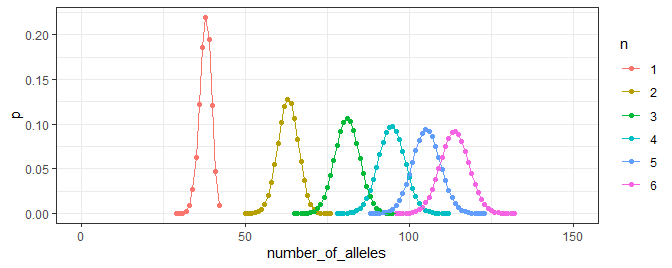# Introduction

In forensic genetics, the number of alleles in a mixed DNA profile is often used to gauge the number of contributors to the mixture. Two statistics are relevant: the total allele count (TAC) across all loci and the maximum allele count (MAC) across the loci. TAC is informative of the number of contributors. MAC can be used as a lower bound: if a mixture has more than $$2n$$ alleles at a locus (MAC exceeds $$2n$$) then there are at least $$n+1$$ mixture donors. For example, if there is a locus with five alleles (MAC=5), then the mixture can not have originated from two donors.

# TAC curves

The numberofalleles package has functionality to compute the probability distribution of the TAC for a given number of contributors. Optionally, subpopulation correction and dropout can be modelled. First, we load the package and read the allele frequencies.

library(numberofalleles)
#>
#> Attaching package: 'numberofalleles'
#> The following object is masked from 'package:stats':
#>
#>     var
library(ggplot2)

package = "numberofalleles"))

gf_loci <- c("D3S1358", "vWA", "D16S539", "CSF1PO", "TPOX", "D8S1179", "D21S11",
"D18S51", "D2S441", "D19S433", "TH01", "FGA", "D22S1045", "D5S818",
"D13S317", "D7S820", "SE33", "D10S1248", "D1S1656", "D12S391",
"D2S1338")

Then, we call the pr_total_number_of_distinct_alleles function for $$n=1,\ldots,6$$ contributors and obtain the TAC curve.

p_by_n <- list()

for (i in 1:6){
p <- pr_total_number_of_distinct_alleles(contributors = paste0("U", seq(i)),
freqs = freqs, loci = gf_loci)

p_by_n[[i]] <- data.frame(n = factor(i),
number_of_alleles = p$noa, p = p$pf)
}

We use ggplot2 to plot the curves.

ggplot(subset(do.call(rbind, p_by_n), p > 1e-5)) +
aes(x = number_of_alleles, y = p, colour = n) +
geom_point() + geom_line() + xlim(0, 150) + theme_bw()